# Adding Grand Total or Average to Pivot Chart

This post will guide you how to add grand total line to a pivot chart in Excel. How do I show grand total line in an existing pivot chart in Excel. How to add average line to pivot chart at top position in Excel. How to add a grand total line on an Excel stacked column pivot chart.

## Adding Grand Total or Average to Pivot Chart

Assuming that you have a list of data in range B1:C5 that contain sales data and you will create a pivot table based on those data, and then create a column chart based on the created pivot table. Now if you want to add average line in the pivot column chart, how to achieve it. Just do the following steps:

#1 select the source data B1:C5, and go to INSER tab, click PivotTable command under Tables group to create a pivot table. And the Create PivotTable dialog will open.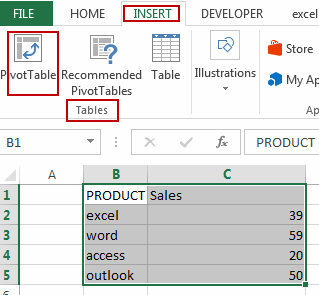#2 select Existing Worksheet radio button, and select one cell as the location. Click Ok button.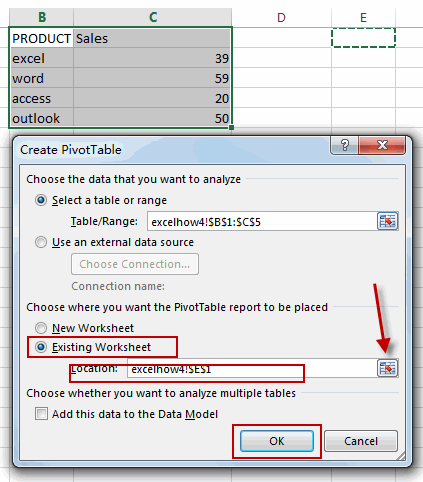#3 checked Fields in Choose fields to add to report section in PivotTable Fields pane. And the pivotTable is created.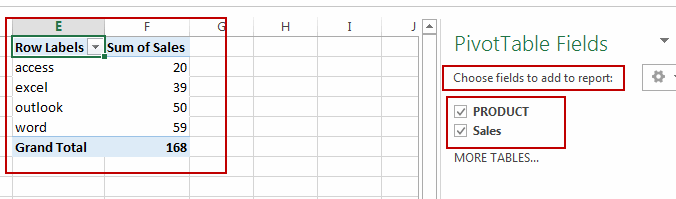#4 select the above pivot table, and go to INSERT tab, click Insert Column Chart command under Charts group. And one Column Chart based on the pivot table is created.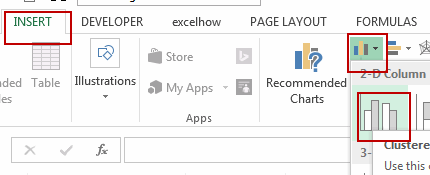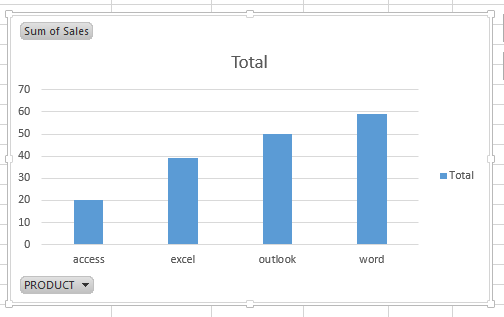#5 select the Sales column in the original data, and right click on it, select Insert from the popup menu list to insert a new column, and type Average in the first cell.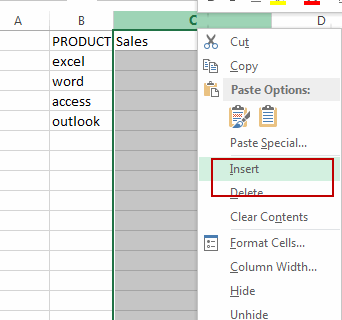#6 type the following formula in the Average column, and then press Enter key, and then drag the AutoFill Handle down to other cells.

=AVERAGE(\$D\$2:\$D\$5)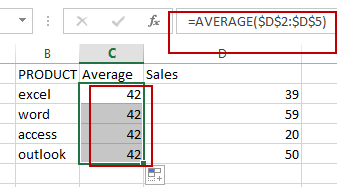#7 select the pivot column chart, and go to ANALYZE tab, click Refresh command under Data group.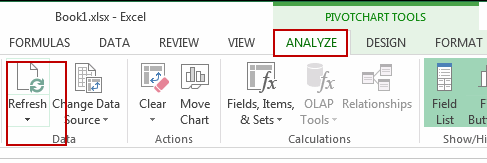#8 The Average field will be displayed in the PivotChart Fields pane, and then checked Average checkbox.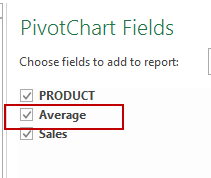#9 select Average Data series in the pivot chart, and select Change Series Chart Type, and the Change Chart Type dialog will open.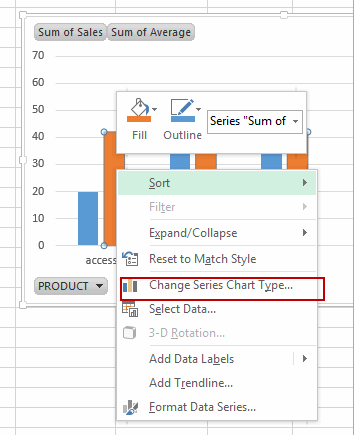#10 click Combo menu in the left pane, and choose the Sum of Average box in the Choose the chart type and axis for your data series section, and select Line as Chart Type. Click OK button.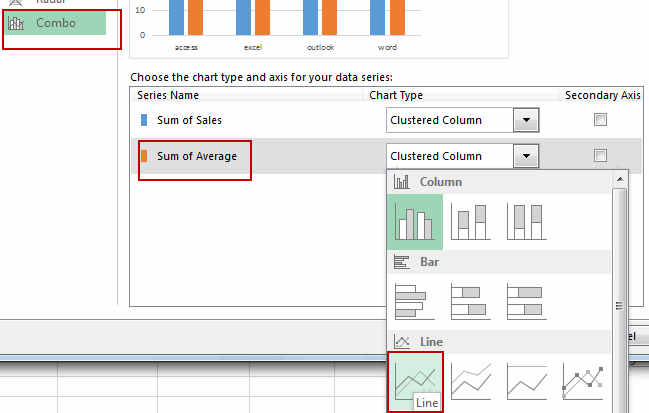#11 the average line has been added into the pivot chart. Let’s see the result.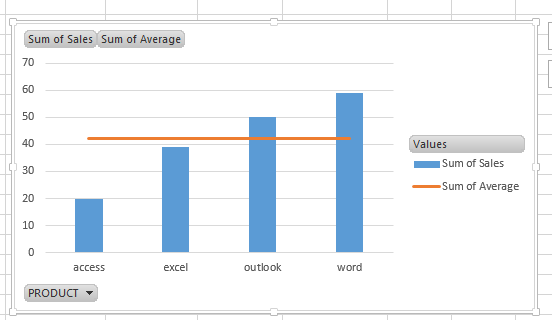Related Posts
If Cell is This Value or That Value

IF function is frequently used in Excel worksheet to return you expect “true value” or “false value” based on the result of logical test. If you want to see if a cell is A or B, and if one of ...

If Value is Greater Than A Certain Value

IF function is frequently used in Excel worksheet to return you expect “true value” or “false value” based on the logical test result. If you want to see if a value in one cell is greater than a specific value, ...

If Cell is Not Blank

IF function is frequently used in Excel worksheet to return you expect “true value” or “false value” based on the result of created logical test. If you want to see if a cell is blank or not, and leave some ...

VBA Macro For VLOOKUP From Another Sheet

In the previous post, you should know that how to fix or remove the #N/A error when using VLOOKUP formula to lookup value from another sheet. And this post will show you how to use VBA code to vlookup data ...

If Cell is Blank

IF function is frequently used in Excel worksheet to return you expect “true value” or “false value” based on the result of created logical test. If you want to see if a cell is blank or not, and leave some ...

If Cell Equals Certain Text String

IF function is frequently used in Excel worksheet to return you expect “true value” or “false value” based on the result of created logical test. If you want to see if cell equals a certain text string like “Win”, you ...

If Cell Contains Either Text1 or Text2

IF function is frequently used in Excel worksheet to return “true value” or “false value” based on the logical test result. If you want to see if cell contains certain substring1 like “abc” or substring2 like “def”, and returns true ...

If Cell Contains Certain Text OR Equals Certain Text

IF cell equals certain text IF function is frequently used in Excel worksheet to return “true value” or “false value” based on the logical test result. If you want to test values to see if they equal certain text like ...

VLOOKUP From Another Sheet Not Working

In the previous post, you should know that how to fix or remove the #N/A error when using VLOOKUP formula to lookup value from another sheet. And this post will show you reasons why your VLOOKUP formula is not working ...

If Cell Begins with One of Three Supplied Characters

If you want to test values to see if they begin with some given specific characters like “x”, ”y”, or “z”, you can create a formula with COUNTIF and SUM functions to return results. EXAMPLE You can see “TRUE” or ...

Sidebar